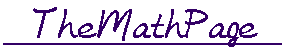# S k i l l   i n   A r i t h m e t i c

HomeIntroduction

Prologue 1     Elementary Addition

Sums less than 10.  Sums equal to 10.
Sums between 10 and 20.  Zero.

Prologue 2     The Multiplication TableLesson 1    Numeration of the Whole Numbers

Units.
Numerals versus numbers.
The names and numerals of the numbers
1 - 999.

 Lesson 2 Reading and Writing Whole Numbers The Powers of 10

How to read a whole number, however large.
Place value numeration.
Expanded form.
Relationship between units of adjacent place value.
How to round off a whole number.
How to multiply a whole number by a power of 10.

Lesson 3    The Meaning of Decimals

Cardinal and ordinal numbers.
Division into equal parts.
The decimal units.
The function of the decimal point.
How to write and how to read a decimal.
Trailing 0's. Leading 0's.
How to compare decimals.

 Lesson 4 Multiplying and Dividing by Powers of 10 The Meaning of Percent

How to multiply or divide a whole number
by a power of 10.
How to multiply or divide a decimal
by a power of 10.
Per centum: For each 100.
How to take 1% of a number.
How to take 10%.

Scientific notation.
The metric system.Lesson 5 More Elementary Addition Mental Calculation

Composing a multiple of 10.
Adding from left to right by place value.
Rounding off.

 Lesson 6 Adding Whole Numbers and Decimals Addition with Regrouping

Written calculations.

 Lesson 7 The Meaning of Subtraction Mental Calculation

What number must we add?
Completing 100.  Rounding off.

 Lesson 8 Subtracting Whole Numbers andDecimals

Written calculation by adding to both numbers.
Written calculation by regrouping, or borrowing.

 Lesson 9 The Meaning of Multiplication Mental Calculation

The multiplier, the multiplicand, the product.
Factors and the order property.
How to multiply whole numbers that end
in 0's.
How to square a number mentally.
Decomposing the multiplier.
Decomposing the multiplicand:
The "distributive" property.
Multiply mentally by rounding off.

 Lesson 10 Multiplying Whole Numbers andDecimals

Written calculation.
How to find a percent of a number.
The area of a rectangle.

Lesson 11    The Meaning of Division

The divisor, the dividend, and the quotient.
How to divide a number into equal parts.
Exact versus inexact division.
The division bar.
Mental calculation:
Decomposing the  dividend.
The divisor a decimal.
Three properties of division.

Lesson 12    Short Division

What is short division?
The dividend a decimal.
How to round off a decimal.
How to express the quotient as a decimal.
The dividend less than the divisor.
Calculator problems.

Lesson 13    Dividing DecimalsLesson 14    Percent with a CalculatorLesson 15    Parts of Natural Numbers 1

What is a natural number?
The definition of a part of a number.
Percent -- parts of 100%.

Lesson 16    Parts of Natural Numbers 2

Finding a part by decomposing.
How to divide by taking a part.
A mixed number of times.
Increase or decrease by a given part.
The percent that means a third.
Even and odd numbers.
Half of any number.
A fourth or 25% of a number.
5% of a number -- 15%.

Lesson 17    Ratio and Proportion

The definition of the ratio of two natural numbers.
Percents are ratios.
What is a proportion?
How to express the ratio of any smaller number to a larger.
Lowest terms.
The theorem of the common divisor.

Lesson 18    Solving Proportions

The theorem of the same multiple.
The Rule of Three.
How to find a percent by the method of proportions.
Mixed ratio.

Lesson 19    Proportionality

Directly proportional.
Inversely proportional.Lesson 20   What is a Fraction?

Common fractions, proper fractions, improper fractions, mixed numbers.
How to place a fraction on the number line.
How to change an improper fraction to a mixed number.
How to change a mixed number to an improper fraction.
How to compare fractions that have equal numerators
How to compare fractions that have equal denominators
"Out of."

 Lesson 21 Unit fractions: Fractions withNumerator 1

Every fraction is a sum of unit fractions.
How to express a whole number as a fraction.
The complement of a proper fraction.
How to subtract a proper fraction from a whole number.

Lesson 22    Equivalent Fractions

How to recognize equivalent fractions.
How to change denominators.
How to simplify or reduce a fraction.
How to clear a fraction of decimals.

 Lesson 23 Lowest common multipleComparing fractions

How to compare fractions by cross-multiplying.
How to compare a fraction to ½.

Lesson 24    Fractions into Decimals

What is a decimal fraction?
Frequent decimals and frequent percents:     Half, quarters, eighths, thirds, fifths.
Exact versus inexact decimals.Lesson 25 Adding and Subtracting Fractions and Mixed Numbers
 Lesson 26 Multiplying Fractions Dividing Fractions

How to multiply a fraction by a whole number.
How to multiply a fraction by a fraction.
Dividing fractions compared to adding them.

 Lesson 27 The Meaning of Multiplying FractionsParts of Fractions

The meaning of multiplying a number by
a fraction.
How to express a fraction as a percent.
How to take a part of a fraction.
The general definition of multiplication.Lesson 28    Percents are Ratios

The ratio to 100%.
Percent increase or decrease. Estimations.

Lesson 29    Percent of a Number

How to find a percent of a number.
What does ½% mean?
A number is a given percent of what other number?

 Lesson 30 What Percent is One Number of Another?

The method of proportions.

Lesson 31    Percent Increase or Decrease

"Difference" problems versus "out of"
problems.
Banking problems.Lesson 32 Prime Numbers and Prime Factorization

The definition of a prime number.
Is there a last prime?

 Lesson 33 Greatest Common Divisor Lowest Common Multiple

How to find the GCD and the LCM of two numbers from their prime factorizations.Appendix 1   Continuous versus Discrete

Appendix 2    Odd and Even Numbers

Square numbers.
Pythagorean triples.
Primes that are 1 more
than a multiple of 4.

Appendix 3    Theoretic Arithmetic

Triangular numbers.
Square numbers.
The sum of consecutive squares.
The sum of consecutive cubes.

Appendix 4    Two Theorems

The distributive property of multiplication.
The order property.